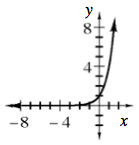### Home > INT3 > Chapter Ch1 > Lesson 1.1.4 > Problem1-60

1-60.

Graph and completely describe the function $f(x) = 4^x$.

Where is the $y$-intercept?
Does it ever cross the $x$-axis?

What are its domain and range?